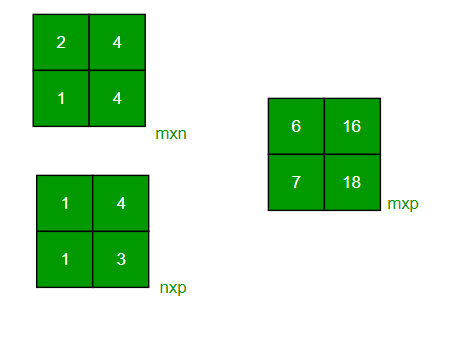GFG App
Open AppBrowser
Continue

# Javascript Program to multiply two matrices

Given two matrices, the task to multiply them. Matrices can either be square or rectangular.

Examples:

```Input : mat1[][] = {{1, 2},
{3, 4}}
mat2[][] = {{1, 1},
{1, 1}}
Output : {{3, 3},
{7, 7}}
Input : mat1[][] = {{2, 4},
{3, 4}}
mat2[][] = {{1, 2},
{1, 3}}
Output : {{6, 16},
{7, 18}}```Multiplication of Square Matrices :
The below program multiplies two square matrices of size 4*4, we can change N for different dimensions.

## Javascript

 ``

Output

```Result matrix is
10 10 10 10
20 20 20 20
30 30 30 30
40 40 40 40```

Time complexity: O(n3). It can be optimized using Strassen’s Matrix Multiplication

Auxiliary Space: O(n2)

Multiplication of Rectangular Matrices :
We use pointers in C to multiply to matrices. Please refer to the following post as a prerequisite of the code.
How to pass a 2D array as a parameter in C?

## Javascript

 ``

Output

```6 16
7 18```

Time complexity: O(n3). It can be optimized using Strassen’s Matrix Multiplication

Auxiliary Space: O(m1 * n2)

Please refer complete article on Program to multiply two matrices for more details!

My Personal Notes arrow_drop_up